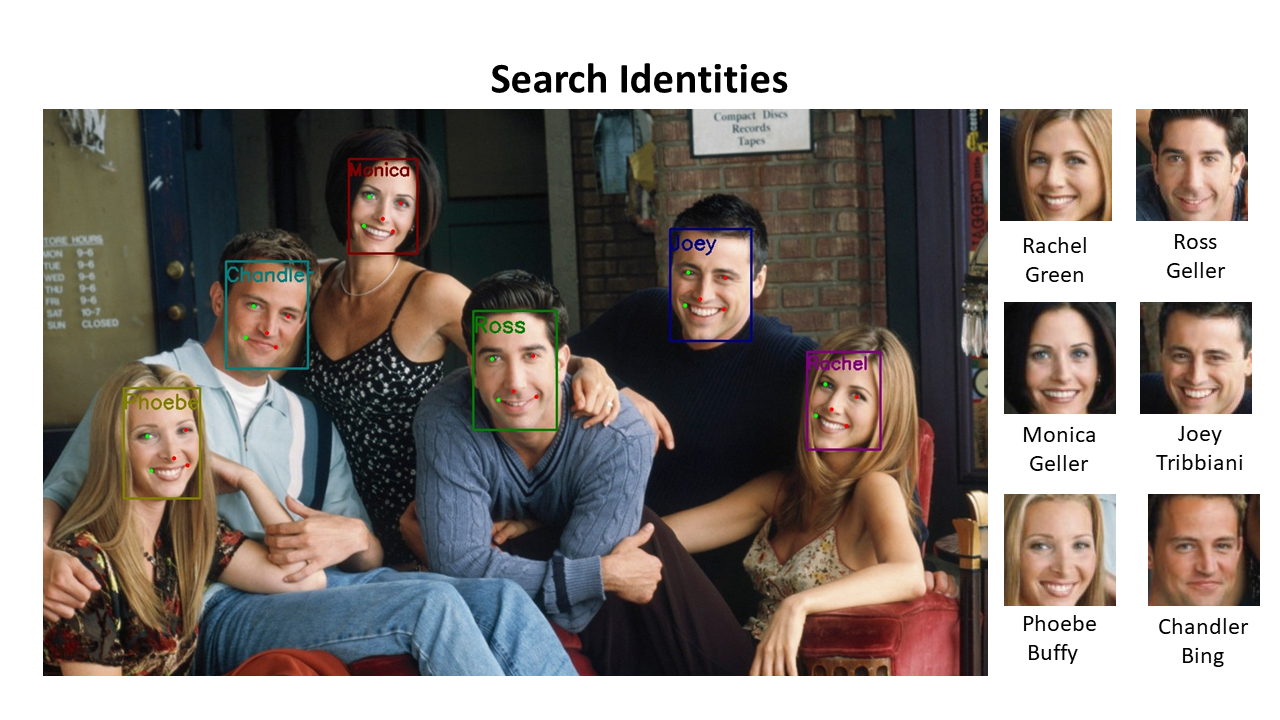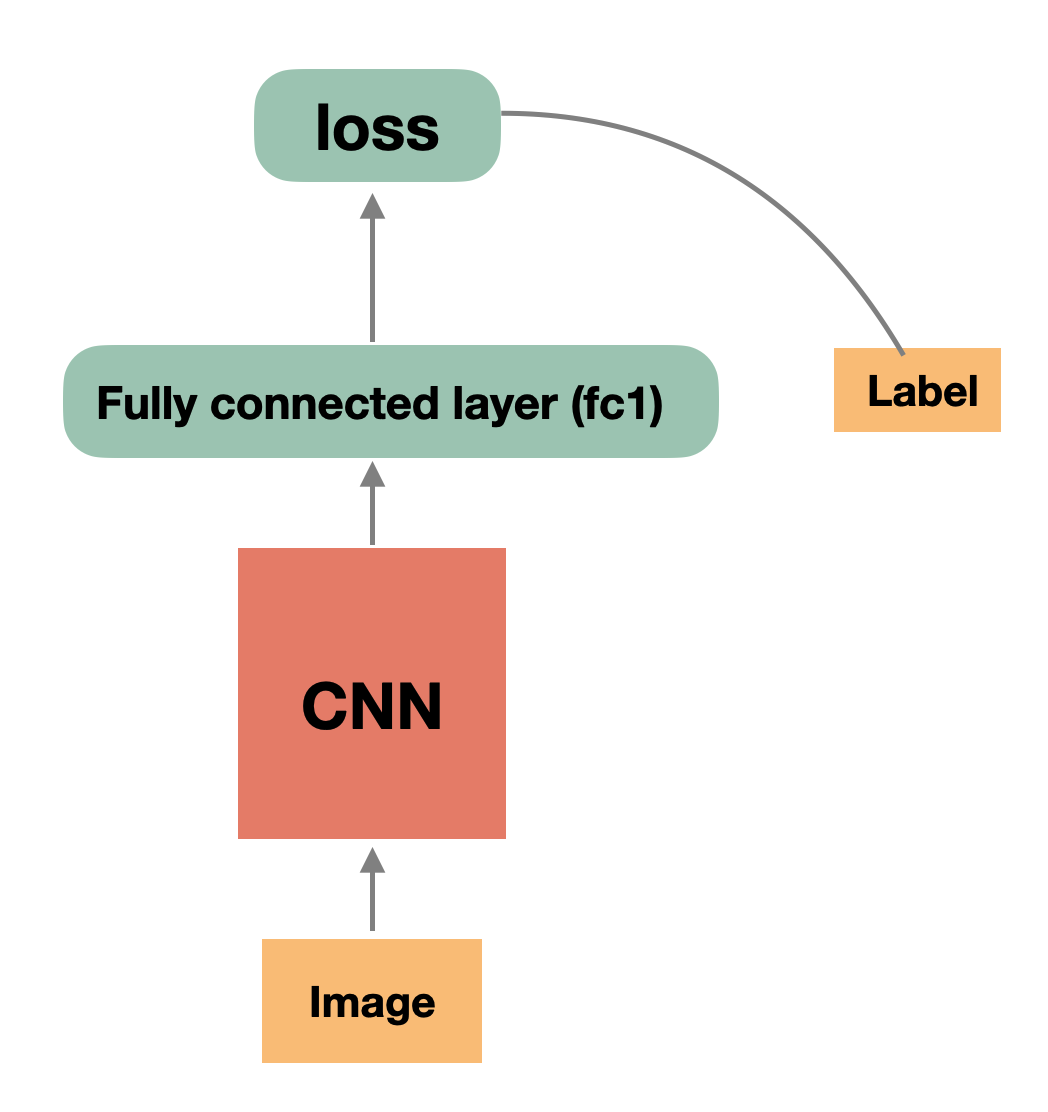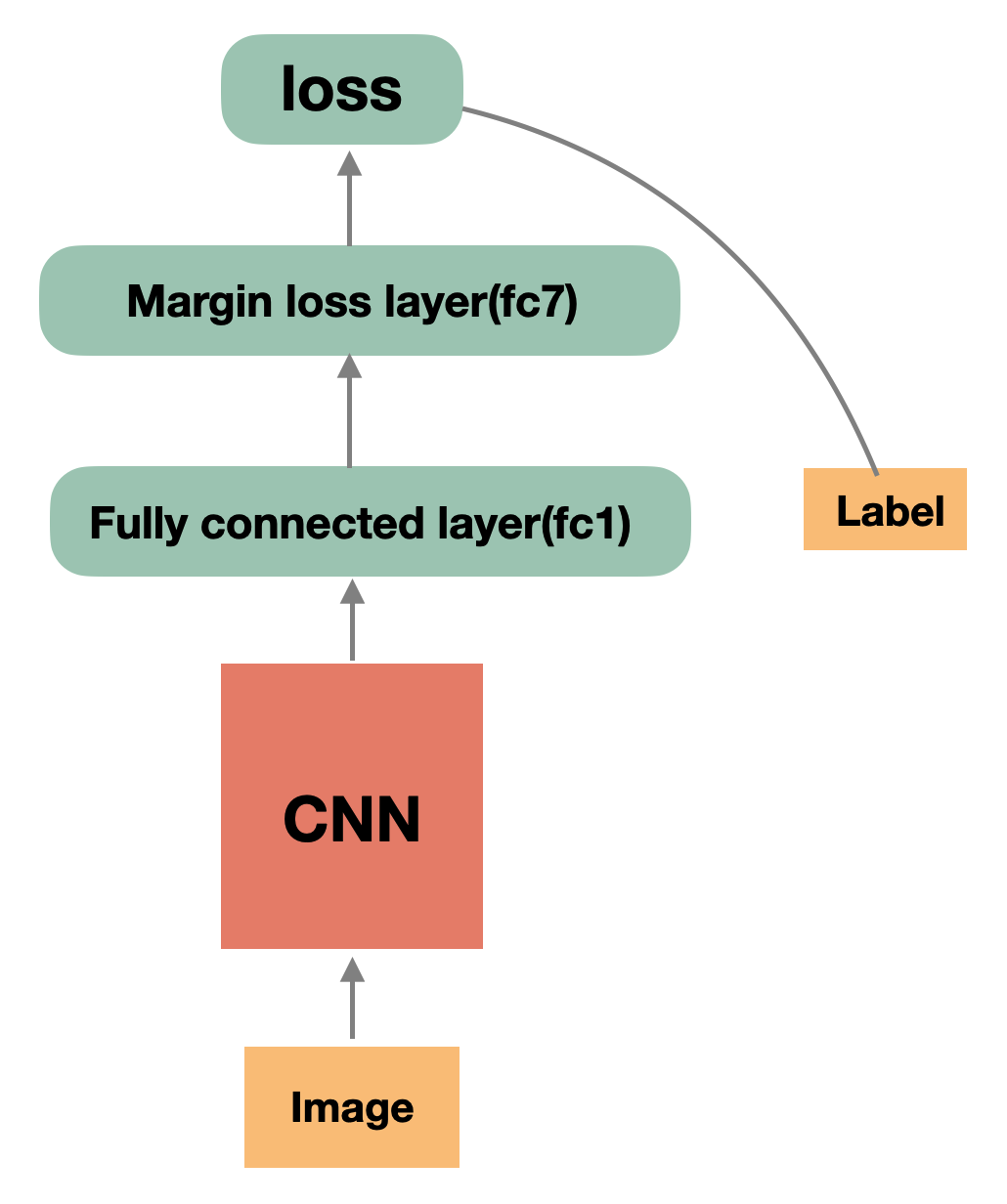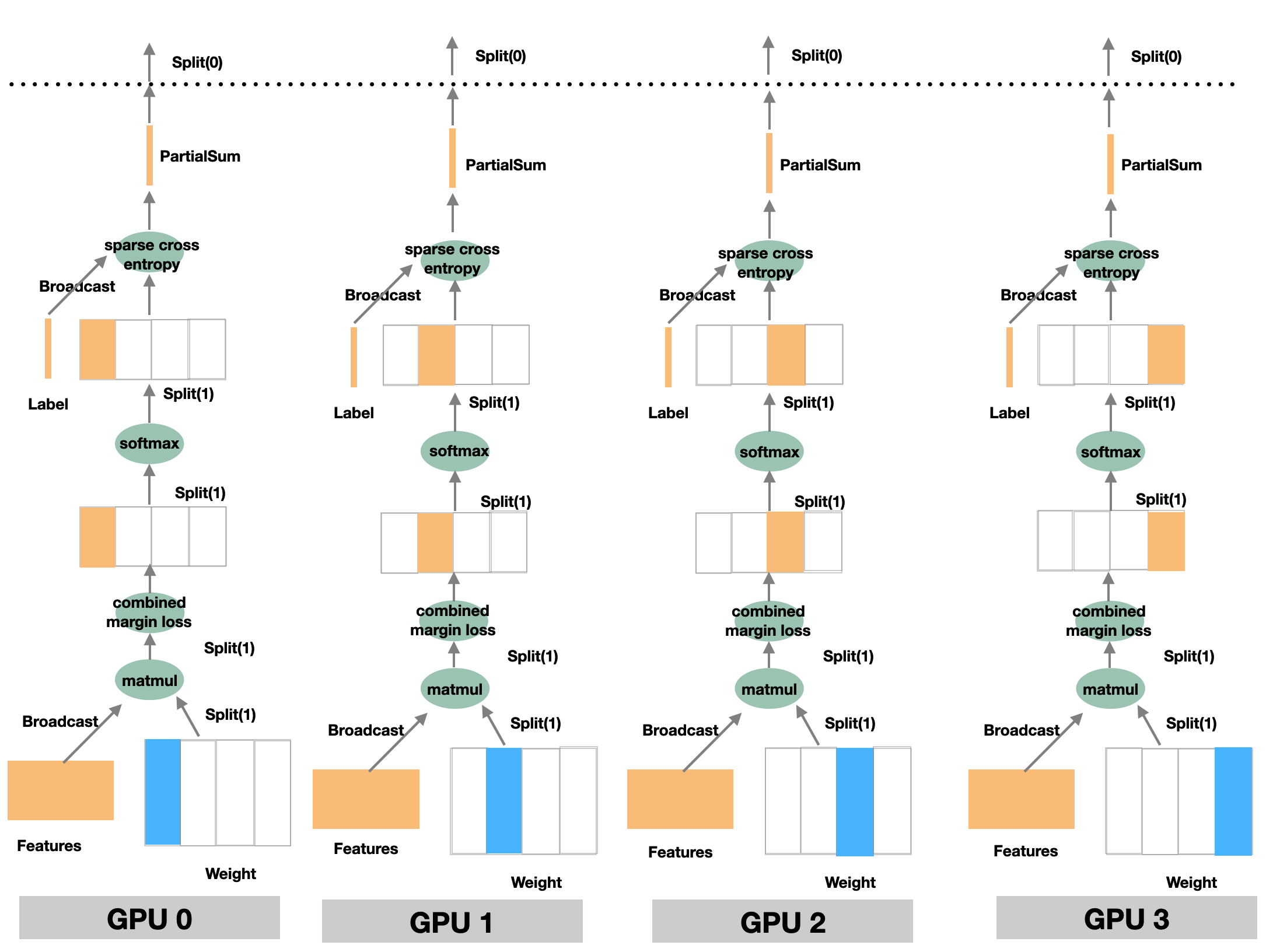InsightFace： 用OneFlow轻松实现超大规模人脸识别模
• 作者：一流科技
• 发表时间：2021-03-04 11:41
• 来源：未知

## 概述OneFlow 作为主打分布式易用和性能的框架，有着去中心化的Actor机制及SBP的抽象，天然对分布式有着良好支持，训练速度快、显存占用低，同样的显卡能支持更大的batch size，从单机多卡拓展到多机分布式训练并不需要复杂的设置。本文基于OneFlow实现了大规模人脸识别的解决方案，该方案可以帮助用户轻松使用数据并行 + 模型并行的混合训练，同时还支持了Partial FC采样技术，理论上支持过亿(ID数)级别的人脸识别。

InsightFace 是基于 MXNet 框架实现的业界主流人脸识别解决方案。相较MXNet的实现方案，基于OneFlow的实现方案在性能方面更是十分优秀，OneFlow在数据并行时速度是其2.82倍；模型并行时速度是其2.45倍；混合并行+Partial fc时速度是其1.38倍。基于OneFlow实现的代码已合并至 insightface的官方仓库，其中包含了数据集制作教程、训练和验证脚本、预训练模型以及和MXNet模型的转换工具。在 The 1:1 verification accuracy on InsightFace Recognition Test (IFRT) 验证集上，Oneflow及MXNet训练模型的精度对比如下：

Framework African Caucasian Indian Asian All
OneFlow 90.4076 94.583 93.702 68.754 89.684
MXNet 90.45 94.60 93.96 63.91 88.23

• 1.大规模人脸识别背景介绍
• 2.OneFlow如何实现大规模人脸识别
• 3.MXNet的大规模人脸识别方案
• 4.数据并行、模型并行解决方案的通信量对比
• 5.Partail FC采样技术
• 6.OneFlow和MXNet实现的性能对比
• 7.总结

## 1.大规模人脸识别背景介绍

### 1.1 面临的问题

#### 简单的网络#### 复杂的MarginLoss

class MarginLoss(object):
""" Default is Arcface loss
"""
def __init__(self, margins=(1.0, 0.5, 0.0), loss_s=64, embedding_size=512):
"""
"""
# margins
self.loss_m1 = margins
self.loss_m2 = margins
self.loss_m3 = margins
self.loss_s = loss_s
self.embedding_size = embedding_size

def forward(self, data, weight, mapping_label, depth):
"""
"""
norm_data = nd.L2Normalization(data)
norm_weight = nd.L2Normalization(weight)
#
fc7 = nd.dot(norm_data, norm_weight, transpose_b=True)
#
mapping_label_onehot = mx.nd.one_hot(indices=mapping_label,
depth=depth,
on_value=1.0,
off_value=0.0)
# cosface
if self.loss_m1 == 1.0 and self.loss_m2 == 0.0:
_one_hot = mapping_label_onehot * self.loss_m3
fc7 = fc7 - _one_hot
else:
fc7_onehot = fc7 * mapping_label_onehot
cos_t = fc7_onehot
t = nd.arccos(cos_t)
if self.loss_m1 != 1.0:
t = t * self.loss_m1
if self.loss_m2 != 0.0:
t = t + self.loss_m2
margin_cos = nd.cos(t)
if self.loss_m3 != 0.0:
margin_cos = margin_cos - self.loss_m3
margin_fc7 = margin_cos
margin_fc7_onehot = margin_fc7 * mapping_label_onehot
diff = margin_fc7_onehot - fc7_onehot
fc7 = fc7 + diff

fc7 = fc7 * self.loss_s
return fc7, mapping_label_onehot


MarginLoss包含m1~m3这3个参数，通过这3个参数的组合来实现cosface loss和arcface loss。而在oneflow中的实现中则更为简便，直接调用flow.combined_margin_loss即可实现MarginLoss系列的功能。

## 2.OneFlow 如何实现大规模人脸识别

### 2.1 整体结构FC层以及具体loss计算的细节，如下图所示：### 2.2 Oneflow实现代码解析

elif config.loss_name == "margin_softmax":
if args.model_parallel:
print("Training is using model parallelism now.")
fc7_data_distribute = flow.distribute.split(1)
fc7_model_distribute = flow.distribute.split(0)
else:
fc1_distribute = flow.distribute.split(0)
fc7_data_distribute = flow.distribute.split(0)
fc7_weight = flow.get_variable(
name="fc7-weight",
shape=(config.num_classes, embedding.shape),
dtype=embedding.dtype,
initializer=_get_initializer(),
regularizer=None,
trainable=trainable,
model_name="weight",
distribute=fc7_model_distribute,
)
if args.partial_fc and args.model_parallel:
print(
"Training is using model parallelism and optimized by partial_fc now."
)
(
mapped_label,
sampled_label,
sampled_weight,
) = flow.distributed_partial_fc_sample(
weight=fc7_weight, label=labels, num_sample=args.total_num_sample,
)
labels = mapped_label
fc7_weight = sampled_weight
fc7_weight = flow.math.l2_normalize(
input=fc7_weight, axis=1, epsilon=1e-10)
fc1 = flow.math.l2_normalize(
input=embedding, axis=1, epsilon=1e-10)
fc7 = flow.matmul(
a=fc1.with_distribute(fc1_distribute), b=fc7_weight, transpose_b=True
)
fc7 = fc7.with_distribute(fc7_data_distribute)
fc7 = (
flow.combined_margin_loss(
fc7, labels, m1=config.loss_m1, m2=config.loss_m2, m3=config.loss_m3
)
* config.loss_s
)
fc7 = fc7.with_distribute(fc7_data_distribute)
else:
raise NotImplementedError

loss = flow.nn.sparse_softmax_cross_entropy_with_logits(
labels, fc7, name="softmax_loss"
)

lr_scheduler = flow.optimizer.PiecewiseScalingScheduler(
base_lr=args.lr,
boundaries=args.lr_steps,
scale=args.scales,
warmup=None
)
flow.optimizer.SGDW(lr_scheduler,
momentum=args.momentum if args.momentum > 0 else None,
weight_decay=args.weight_decay
).minimize(loss)

return loss


fc1_distribute = flow.distribute.broadcast()     #  表示SBP为B，广播，用于全量数据的同步

fc7_model_distribute = flow.distribute.split(0)  #  表示SBP为S0，表示模型并行且按第0维切割
# （FC层为dense层，在框架实现上会对dense层模型做转置，model_distribute设Split(0)代表模型在第1维分割，SBP属性相当于Split(1)

fc7_data_distribute = flow.distribute.split(1)   #  表示SBP为S1，表示数据并行且按第1维切割（和模型并行保持一致）


• 1.首先，通过flow.matmul()进行矩阵乘法(fc1层->fc7层的前向传播)，并设置其结果的SBP属性为fc7_data_distribute。
• 2.然后，通过flow.combined_margin_loss()完成fc7层的前向传播，由于其SBP属性并没有改变，故继续设置其SBP属性为fc7_data_distribute。
• 3.最后，直接调用flow.nn.sparse_softmax_cross_entropy_with_logits()即可完成求softmax交叉墒损失，至此完成了整个过程前向+loss计算，之后的反向过程、梯度更新、学习率更新等将由后面的flow.optimizer自动完成。

### 2.3 模型并行的技术细节

#### 模型并行下的softmax

• 公式中涉及到的求 max 和求 sum 的运算均需要全局的信息
• 但是每个 GPU 设备上只有部分的模型

#### sparse_softmax_cross_entropy_with_logits 的实现细节

reduce max

reduce max的作用是按列取出最大，以形状为 (3, 3) 的数据块为例，其效果为：

[5, 4, 2]                 
[4, 3, 3]  -reduce max->  
[1, 9, 8]                 


sub

exp

reduce sum

div

sparse cross entropy 的输入有两个：

• 前一层 softmax 的输出，SBP 属性为 Split(1)

OneFlow 会根据 sparse cross entropy 输入的 SBP 属性，自动推导出其输出的 SBP 属性为 Partial sum。 sparse_cross_entropy 的输出，经过 Partial sum 后，再广播到各个卡，同 softmax 的情况类似，因为数据块已经经过减小，因此大大减少了通信量。

#### OneFlow 中的代码实现

labels = flow.parallel_cast(labels, distribute =  flow.distribute.broadcast())
embedding = flow.parallel_cast(embedding, distribute = flow.distribute.broadcast())

fc7 = flow.layers.dense(
inputs=embedding,
units=args.class_num,
model_distribute=flow.distribute.split(0),
) #dense中模型会做转置，model_distribute设Split(0)代表模型在第1维分割，SBP属性为Split(1)

loss = flow.nn.sparse_softmax_cross_entropy_with_logits(
labels, fc7.with_distribute(flow.distribute.split(1)), name="softmax_loss"
)


## 3.MXNet的大规模人脸识别方案

• 数据+模型的混合并行下网络、梯度、数据的处理；
• 混合并行模式下常规的算子如gloss.SoftmaxCrossEntropyLoss 无法正常工作的问题。

MXNet的解决方案为采用horovod、类似numpy的mxnet.ndarray对矩阵进行手动切分计算和手写softmax交叉熵相关的代码，整体实现相对复杂。下面我们将对MXNet实现方案的实现流程做简单讲解，包含前向、反向和Marginloss相关部分。

### 3.1 MXNet实现代码解析

train_module.fit(train_data_iter,
optimizer_params=backbone_kwargs,
initializer=mx.init.Normal(0.1),
batch_end_callback=call_back_fn)


• 通过 forward_backward（）来进行前向、反向的过程，并计算出待更新的梯度；
• 通过 update() 调用 optimizer 完成对模型、学习率等参数的更新。

def forward_backward(self, data_batch):
"""A convenient function that calls both forward and backward.
"""
total_feature, total_label = self.forward(data_batch, is_train=True)
self.backward_all(total_feature, total_label)


#### 前向

def forward(self, data_batch, is_train=None):
self.backbone_module.forward(data_batch, is_train=is_train)
if is_train:
self.num_update += 1
fc1 = self.backbone_module.get_outputs()
label = data_batch.label

total_features = self.allgather(tensor=fc1,
name='total_feature',
shape=(self.batch_size * self.size,
self.embedding_size),
dtype='float32',
context=self.gpu)
total_labels = self.allgather(tensor=label,
name='total_label',
shape=(self.batch_size *
self.size, ),
dtype='int32',
context=self.cpu)
return total_features, total_labels
else:
return None


#### 反向

 def backward_all(
self,
total_feature,
total_label,
):
# get memory bank learning rate
self.memory_lr = self.memory_optimizer.lr_scheduler(self.num_update)

total_feature.shape)
self.loss_cache = self.get_ndarray(self.gpu, 'loss_cache', )

self.loss_cache[:] = 0

if not bool(config.sample_ratio - 1):
else:

self.loss_cache = loss

self.rank:self.batch_size * self.rank +
self.batch_size]


• 第1部分，对Marginloss层的处理集中放在了backward()和backward_sample()部分，二者区别在于backward()对应的是sample_ratio=1.0时的反向（不使用Partial fc进行采样）；backward_sample()对应使用Partial fc进行采样时的反向过程。
• 第2部分，实现CNN网络的反向，即：self.backbone_module.backward

#### Marginloss

def backward(self, total_feature, label):
memory_bank = self.memory_bank
assert memory_bank.num_local == memory_bank.num_sample, "pass"

_data = self.get_ndarray2(self.gpu, "data_%d" % self.rank,
total_feature)

# Convert label
_label = self.get_ndarray2(self.gpu, 'label_%d' % self.rank, label)
_label = _label - int(self.rank * memory_bank.num_local)
_fc7, _one_hot = self.fc7_model.forward(_data,
memory_bank.weight,
mapping_label=_label,
depth=memory_bank.num_local)

# Sync max
max_fc7 = nd.max(_fc7, axis=1, keepdims=True)
max_fc7 = nd.reshape(max_fc7, -1)

total_max_fc7 = self.get_ndarray(context=self.gpu,
name='total_max_fc7',
shape=(max_fc7.shape, self.size),
dtype='float32')
total_max_fc7[:] = 0
total_max_fc7[:, self.rank] = max_fc7
hvd.allreduce_(total_max_fc7, average=False)

global_max_fc7 = self.get_ndarray(context=self.gpu,
name='global_max_fc7',
shape=(max_fc7.shape, 1),
dtype='float32')
nd.max(total_max_fc7, axis=1, keepdims=True, out=global_max_fc7)

# Calculate exp(logits)

# Calculate sum
global_sum_fc7 = hvd.allreduce(sum_fc7, average=False)

# Calculate prob

# Calculate loss
tmp = nd.sum(tmp, axis=1, keepdims=True)
tmp = self.get_ndarray2(self.gpu, 'ctx_loss', tmp)
tmp = hvd.allreduce(tmp, average=False)
global_loss = -nd.mean(nd.log(tmp + 1e-30))

# Backward

# Update center
self.memory_optimizer.update(weight=memory_bank.weight,
state=memory_bank.weight_mom,
learning_rate=self.memory_lr)



_fc7, _one_hot = self.fc7_model.forward(_data,
memory_bank.weight,
mapping_label=_label,
depth=memory_bank.num_local)


## 4.数据并行、模型并行解决方案的通信量对比

### 4.1 数据并行通信量

2 * emb_size * 10000000 * (P - 1)

### 4.2 混合并行通信量

• 1.CNN 网络得到的人脸特征由 Split(0)->Broadcast 的传输，数据块大小为 (batch_size, emb_size)
• 2.label 由 Split(0)->Broadcast 的传输，数据块大小为 (batch_size, 1)
• 3.Softmax 计算过程中，每卡 Split(1) 切分数据的 max 值由 Partial max->Broadcast 的传输，数据块大小为(batch_size, 1)；每卡 Split(1) 切分数据的 sum 值由 Partial sum->Broadcast 的传输，数据块大小为(batch_size, 1)
• 4.最终算出 loss 后的通信，数据块大小为 (batch_size, 1)

(batch_size * emb_size + 6 * batch_size) * (P - 1)

### 4.3 对比总结

2 * emb_size * 10000000 * (P - 1) VS batch_size * （emb_size + 6) * (P - 1)

## 5.Partial FC采样技术

fc7 = flow.matmul(
a=fc1.with_distribute(fc1_distribute), b=fc7_weight, transpose_b=True
)


(batch_size_per_device * P, emb_size)

OneFlow中也支持了Partial FC采样的功能，只需调用flow.distributed_partial_fc_sample算子即可对权重矩阵、标签label进行采样。使用Partial FC采样的主要代码如下：

(mapped_label, sampled_label, sampled_weight) = flow.distributed_partial_fc_sample(
weight=fc7_weight, label=labels, num_sample= num_sample)


## 6.OneFlow和MXNet实现的性能对比

1.在相同batch size下，吞吐率更高，训练速度更快且多卡时，性能损失较小，更接近线性加速比。其中：

• 数据并行时，1836.8 vs 650.8(samples/s)，速度是MXNet的2.82倍
• 模型并行时，1854.15 vs 756.96(samples/s)，速度是MXNet的2.45倍
• 混合并行+Partial fc时(4机32GPU)，6931.6 vs 5008.7(samples/s)，速度是MXNet的1.38倍，且加速比28.1 vs 22.8

2.对GPU显存的管理水平和利用率更高，在相同硬件配置下（gpu显存固定时）支持跑更大的batch size

• 模型并行时，115 vs 96，较MXNet提升20%
• 混合并行+Partial fc时，115 vs 96，较基于MXNet实现的Partial fc提升20%

3.支持更大规模的人脸ID类别数

• 混合并行+Partial fc时，单机支持的最大人脸ID数(num classes)：1350万 vs 1200万，较基于MXNet的Partial fc提升12.5%

### 6.1 数据并行

• dataset: emore
• backbone:resnet100

FP32 & Batch size = 64

OneFlow   MXNet
node_num gpu_num_per_node samples/s speedup samples/s speedup
1 1 245.0 1 241.82 1
1 4 923.23 3.77 655.56 2.71
1 8 1836.8 7.50 650.8 2.69

### 6.2 模型并行

• dataset: emore
• backbone:resnet100

FP32 & Batch size = 64

OneFlow   MXNet
node_num gpu_num_per_node samples/s speedup samples/s speedup
1 1 245.29 1 233.88 1
1 4 938.83 3.83 651.44 2.79
1 8 1854.15 7.55 756.96 3.24

### 6.3 混合并行 + Partial FC(sample ratio = 0.1)

#### emore数据集

• dataset: emore
• backbone:resnet100

FP32 & Batch size = 64

OneFlow   MXNet
node_num gpu_num_per_node samples/s speedup samples/s speedup
1 1 246.45 1 218.84 1
1 4 948.96 3.85 787.07 3.6
1 8 1872.81 7.60 1423.12 6.5
2 8 3540.09 14.36 2612.65 11.94
4 8 6931.6 28.13 5008.72 22.89

#### glint360k数据集

• dataset: glint360k
• backbone:resnet100

FP32 & Batch size = 64

OneFlow   MXNet
node_num gpu_num_per_node samples/s speedup samples/s speedup
1 1 245.12 1 194.01 1
1 4 945.44 3.86 730.29 3.76
1 8 1858.57 7.58 1359.2 7.01

### 6.4 Max batch size per device

OneFlow   MXNet
dataset max batch size per device   max batch size per device
glint360k 115   96

### 6.5 Max num classes

mode node_num gpu_num_per_node max num classes(OneFlow)   max num classes(MXNet)
AMP 1 1 2000000   180 0000
AMP 1 8 13500000   1200 0000

## 7.总结

• 扩展性强、可以轻松支持千万、最大支持亿级别的类别 ID 数的人脸识别模型。
• 不增加额外通信，在 FC 层甚至大大降低了通信量，训练速度更快，更近线性加速比。
• 速度和灵活性上的优势得益于SBP的抽象以及以分布式易用性为出发点的系统设计，OneFlow实现数据并行/模型并行方案只需要很少量的代码，无需对dataset、optimizer、model等做额外包装、无需使用horovod等第三方工具，对算法开发工程师来说更为友好。

## 8.致谢

OneFlow复现、调试Insightface的过程中，需要特别感谢Insightface项目的发起人过佳以及Partial fc的作者格灵深瞳的安翔。首先感谢两位提供了如此高效的大规模人脸识别方案，其次，在OneFlow方案实现过程中，他们给出了耐心细致的指导，在数据集、测试方面也给予了大力支持，衷心感谢！